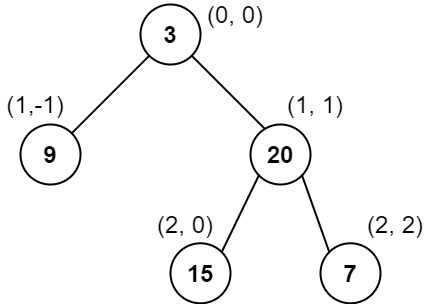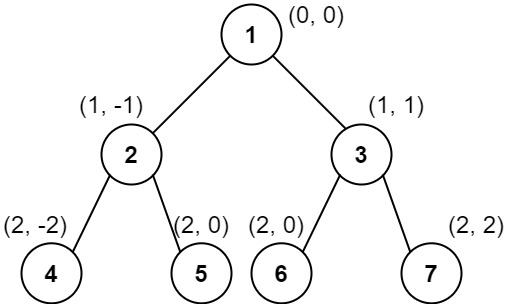# Vertical Order Traversal of Binary Tree LeetCode Solution

Difficulty Level Hard
Binary Tree categories - Medium Depth First Search Hashing tiktok TreeViews 198

## Problem Statement

Vertical Order Traversal of Binary Tree LeetCode Solution says – Given the `root` of a binary tree, calculate the vertical order traversal of the binary tree.

For each node at position `(row, col)`, its left and right children will be at positions `(row + 1, col - 1)` and `(row + 1, col + 1)` respectively. The root of the tree is at `(0, 0)`.

The vertical traversal of a binary tree is a list of top-to-bottom orderings for each column index starting from the leftmost column and ending on the rightmost column. There may be multiple nodes in the same row and same column. In such a case, sort these nodes by their values.

Return the vertical order traversal of the binary tree.

Example 1:Input:

``` root = [3,9,20,null,null,15,7]
```

Output:

``` [,[3,15],,]
```

Explanation:

```Column -1: Only node 9 is in this column.
Column 0: Nodes 3 and 15 are in this column in that order from top to bottom.
Column 1: Only node 20 is in this column.
Column 2: Only node 7 is in this column.```

Example 2:Input:

``` root = [1,2,3,4,5,6,7]
```

Output:

``` [,,[1,5,6],,]
```

Explanation:

```Column -2: Only node 4 is in this column.
Column -1: Only node 2 is in this column.
Column 0: Nodes 1, 5, and 6 are in this column.
1 is at the top, so it comes first.
5 and 6 are at the same position (2, 0), so we order them by their value, 5 before 6.
Column 1: Only node 3 is in this column.
Column 2: Only node 7 is in this column.
```

Example 3:Input:

``` root = [1,2,3,4,6,5,7]
```

Output:

``` [,,[1,5,6],,]
```

Constraints:

• The number of nodes in the tree is in the range `[1, 1000]`.
• `0 <= Node.val <= 1000`

## Algorithm:

### Idea:

• In order to find Vertical Order Traversal of Binary Tree. First, we will focus on the index of all the elements then we will create a list and hashmap and Stores all the value of the same index into a list within the hashmap then sort the hashmap and at last we will return that list as our answer.

### Approach:

• First, we will make one queue and one answer list, and one Hashmap after that we will use level order traversal in order to achieve the answer.
• Then we will make one tuple pair i.e (root,0) [0 – Index of root element] after that when we will traverse level-wise we decrease the value of the index by 1 in the case of root.left element and Increase the value of the index by 1 in case root. right element.
• Also in each iteration, we’ll check if the index already exists in Hashmap, if it exists then we will append the value of the index into the index key otherwise if not then create a new key i.e Index and store the value as a list of root.val.
• After that, we will sort the hashmap and add all lists into the answer list and Finally return that list as our answer.
• Hence, we will find the Vertical Order Traversal of the Binary Tree.## Code:

### Vertical Order Traversal of a Binary Tree Python Leetcode Solution:

```class Solution:
def verticalTraversal(self, root: Optional[TreeNode]) -> List[List[int]]:

queue = [(root,0)]
dic = {}
ans = []

while(queue):
k = queue
queue = []
for v,i in k:

if i in dic:
dic[i].append(v.val)

else:
dic[i] = [v.val]

if v.left:
queue.append((v.left,i-1))

if v.right:
queue.append((v.right,i+1))

queue.sort(key = lambda x:(x,x.val))

for i in sorted(dic):
ans.append(dic[i])

return ans
```

### Vertical Order Traversal of a Binary Tree Java Leetcode Solution:

```class Solution {
public List<List<Integer>> verticalTraversal(TreeNode root) {

class Group{
TreeNode treeNode;
int dis;
int vd;
Group(TreeNode treeNode, int dis, int vd){
this.treeNode = treeNode;
this.dis = dis;
this.vd = vd;
}
}
List<List<Integer>> ans = new ArrayList();
if( root == null)   return ans;
Map<Integer,List< Group>> dic = new HashMap();
List<Group > list = new ArrayList();
int minDis = Integer.MAX_VALUE;
int maxDis = Integer.MIN_VALUE;

while( ! queue.isEmpty() ){
Group group = queue.poll();

minDis = Math.min(minDis, group.dis);
maxDis = Math.max(maxDis, group.dis);

if( group.treeNode.left !=null ) {
}
if( group.treeNode.right !=null){
}

if( dic.containsKey(group.dis)){
list = dic.get(group.dis);
dic.put(group.dis,new ArrayList(list ));
list.clear();
}
else{
dic.put(group.dis, new ArrayList(list));
list.clear();
}
}

int index = 0;
for(int d = minDis; d <= maxDis; d++ ){
list =  dic.get(d);
Collections.sort(list, (a,b) -> {

if( a.vd == b.vd)   return a.treeNode.val - b.treeNode.val ;
else
return a.vd - b.vd;

} );

for(Group g : list){
}

index++;
}
return ans;
}
}
```

## Complexity Analysis of Vertical Order Traversal of a Binary Tree Leetcode Solution:

### Time complexity:

The Time Complexity of the above solution is O(nlogn) where n = the number of nodes in the Binary Tree. We have traversed the whole array and sorted the hashmap.

### Space complexity:

The Space Complexity of the above solution is O(n) since we have created a queue that has a maximum size of n.

Translate »